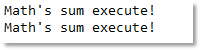# java中多态基础语法

1. 如果当前在 Flash 界面下弹出的就是 AS 3 的帮助文档；
2. 如果当前在 Word 下弹出的就是 Word 帮助；
3. 如果当前在 Windows 下弹出的就是 Windows 帮助和支持。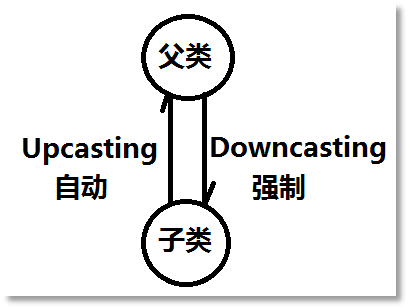``````public class Animal {
public void move(){
System.out.println("Animal move!");
}
}``````

``````public class Cat extends Animal{
//方法覆盖
public void move(){
System.out.println("走猫步！");
}
//子类特有
public void catchMouse(){
System.out.println("抓老鼠！");
}
}``````

``````public class Bird extends Animal{
//方法覆盖
public void move(){
System.out.println("鸟儿在飞翔！");
}
//子类特有
public void sing(){
System.out.println("鸟儿在歌唱！");
}
}``````

``````public class Test01 {
public static void main(String[] args) {
//创建Animal对象
Animal a = new Animal();
a.move();
//创建Cat对象
Cat c = new Cat();
c.move();
//创建鸟儿对象
Bird b = new Bird();
b.move();
}
}``````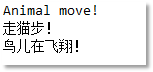``````public class Test02 {
public static void main(String[] args) {
Animal a1 = new Cat();
a1.move();
Animal a2 = new Bird();
a2.move();
}
}``````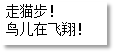``````public class Test03 {
public static void main(String[] args) {
Animal a = new Cat();
a.catchMouse();
}
}``````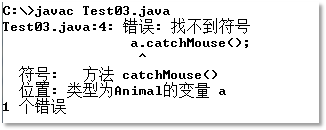``````public class Test04 {
public static void main(String[] args) {
//向上转型
Animal a = new Cat();
//向下转型：为了调用子类对象特有的方法
Cat c = (Cat)a;
c.catchMouse();
}
}``````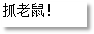``````public class Test05 {
public static void main(String[] args) {
Animal a = new Bird();
Cat c = (Cat)a;
}
}``````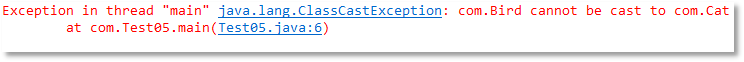instanceof运算符的语法格式是这样的：

(引用 instanceof 类型)

instanceof运算符的运算结果是布尔类型，可能是true，也可能是false，假设(c instanceof Cat)结果是true则表示在运行阶段c引用指向的对象是Cat类型，如果结果是false则表示在运行阶段c引用指向的对象不是Cat类型。有了instanceof运算符，向下转型就可以这样写了：

``````public class Test05 {
public static void main(String[] args) {
Animal a = new Bird();
if(a instanceof Cat){
Cat c = (Cat)a;
c.catchMouse();
}
}
}``````

以上程序运行之后不再发生异常，并且什么也没有输出，那是因为if语句的条件并没有成立，因为在运行阶段a引用指向的对象不是Cat类型，所以(a instanceof Cat)是false，自然就不会进行向下转型了，也不会出现ClassCastException异常了。在实际开发中，java中有这样一条默认的规范需要大家记住：在进行任何向下转型的操作之前，要使用instanceof进行判断，这是一个很好的编程习惯。就像下面的代码：

``````public class Test05 {
public static void main(String[] args) {
Animal a = new Bird();
if(a instanceof Cat){
Cat c = (Cat)a;
c.catchMouse();
}else if(a instanceof Bird){
Bird b = (Bird)a;
b.sing();
}
}
}``````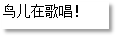• 继承
• 方法覆盖
• 父类型引用指向子类型对象

``````public class OverrideTest {
public static void main(String[] args) {
Math.sum();
MathSubClass.sum();
}
}``````

``````public class Math{
public static void sum(){
System.out.println("Math's sum execute!");
}
}``````

``````public class MathSubClass extends Math{
//尝试覆盖从父类中继承过来的静态方法
public static void sum(){
System.out.println("MathSubClass's sum execute!");
}
}``````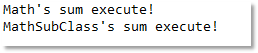``````public class OverrideTest {
public static void main(String[] args) {
Math m = new MathSubClass();
m.sum();
m = null;
m.sum();
}
}``````